05 217 385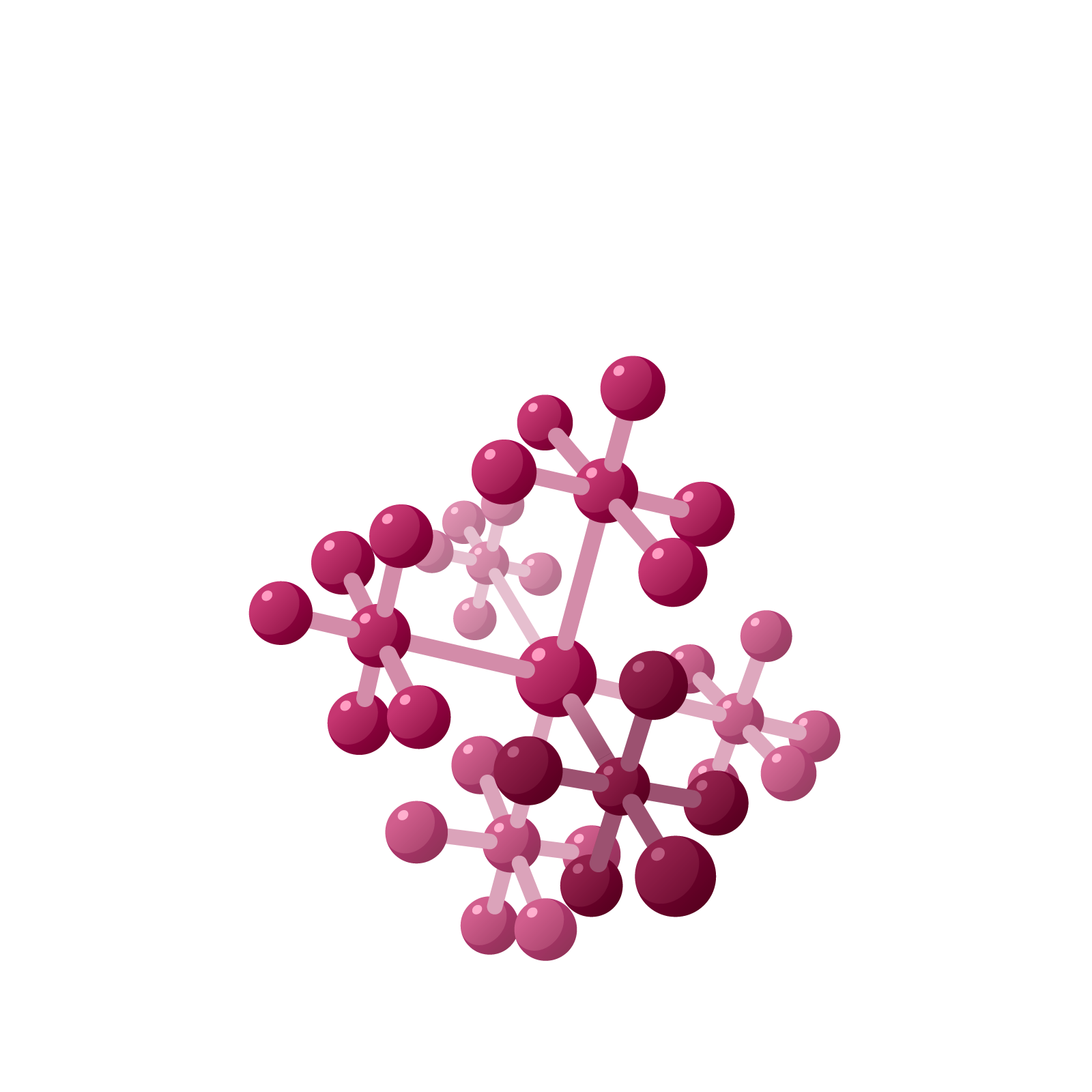# Station  11DSubtractionNapier did not introduce the notion of an antidot, but suggested performing subtraction this way instead.To compute $106-49$ , say, represent the larger number on the second row of the board and the smaller number on the bottom row.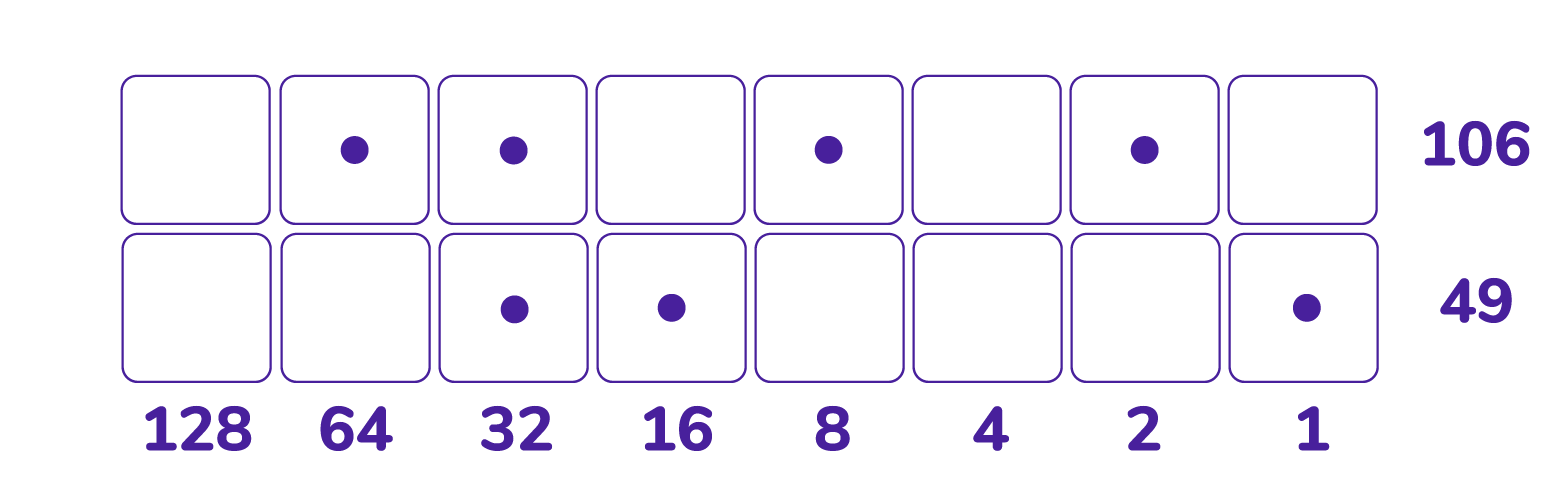Starting at the left of the second row, perform unexplosions so that each dot in the bottom row has at least one dot above it.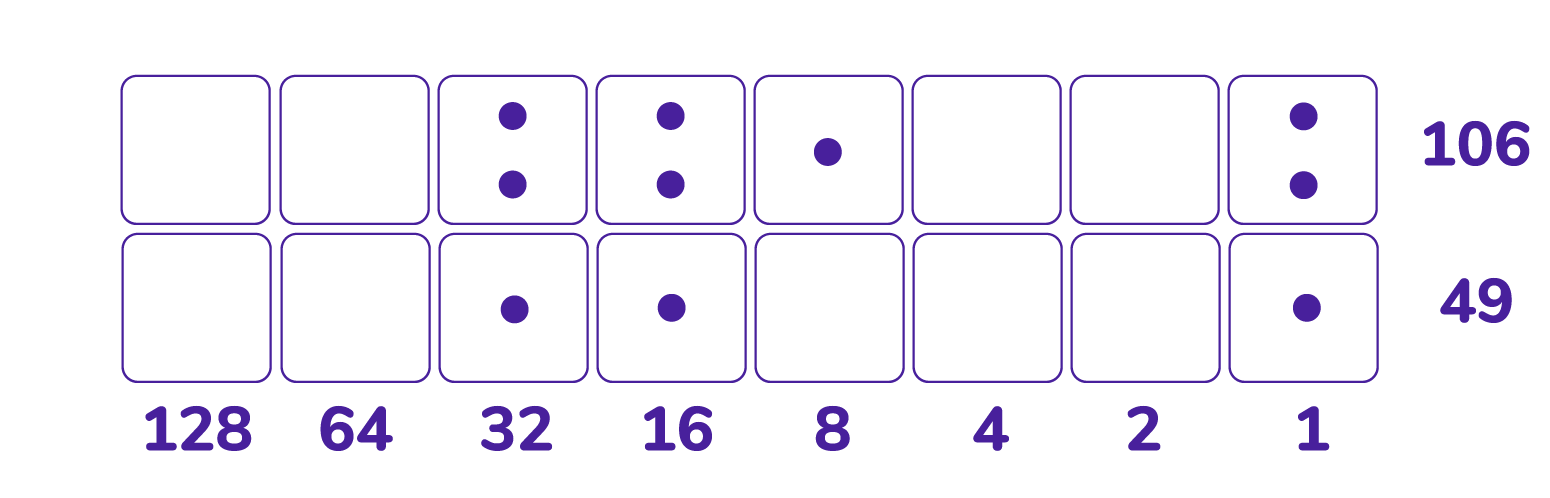Now subtract dots from the second row, one for each dot that sits on the first row. We see the answer $57$ appear.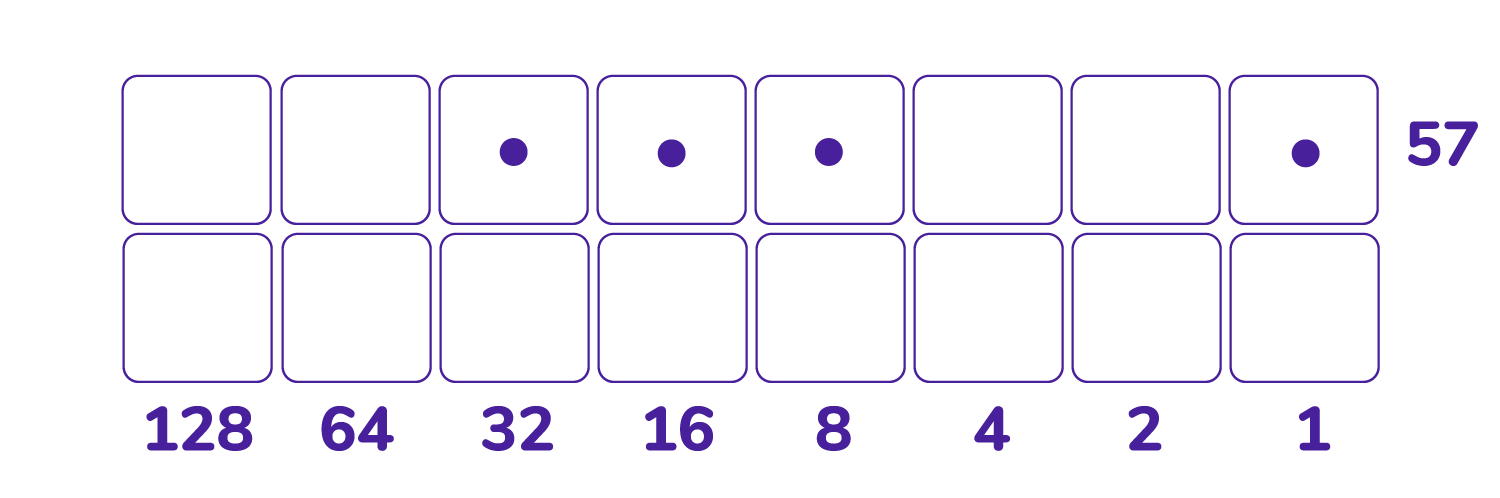Question: The picture below shows how we performed subtraction in the $1 \leftarrow 2$ machine using antidots. Can you see a correlation of the two approaches?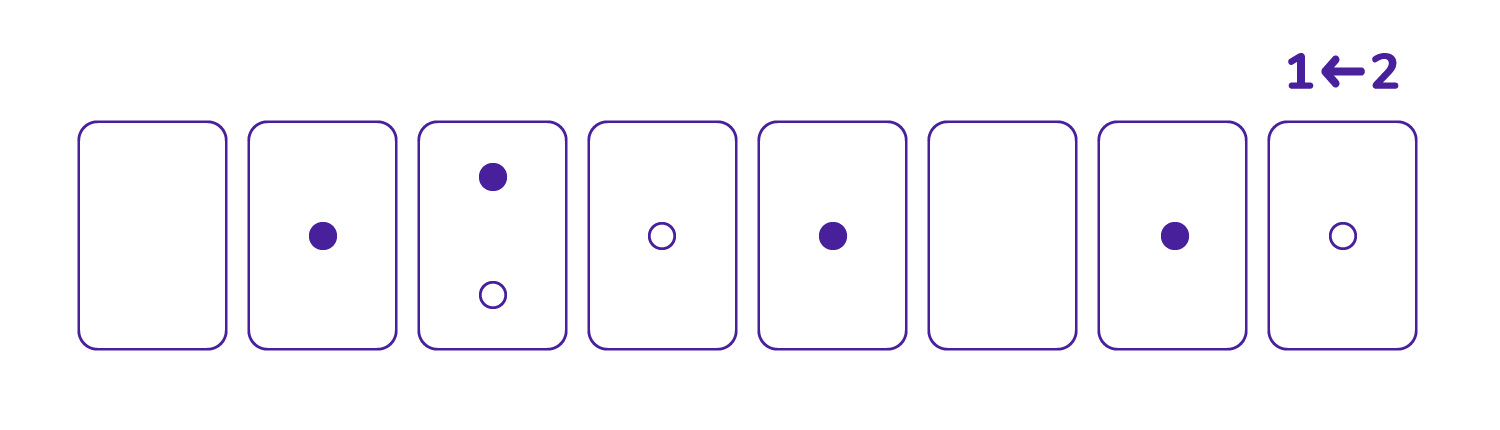Question: Consider a $1 \leftarrow 2$ machine fully loaded as shown.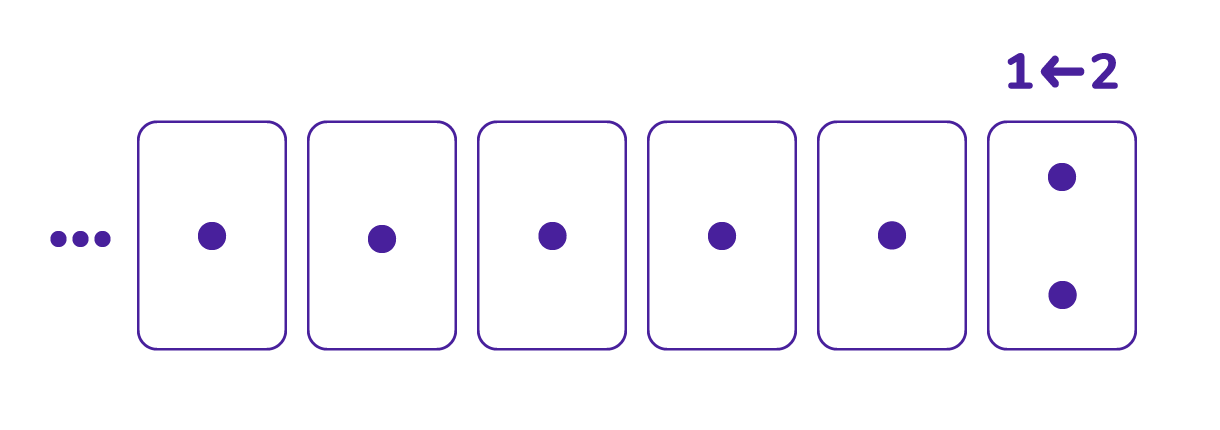Do you see if you perform all the explosions, all the dots disappear? This shows that, in some sense, the infinitely long base-two number $\cdots 111112$ represents the number zero. (See the chapter on Some Unusual Mathematics for Unusual Numbers for more on this.)

This means we can add $\cdots 11112$ to a picture of a negative number and not change the number. For example, we see that another representation of $-49$ in a $1 \leftarrow 2$ machine is $\cdots 11111001111$.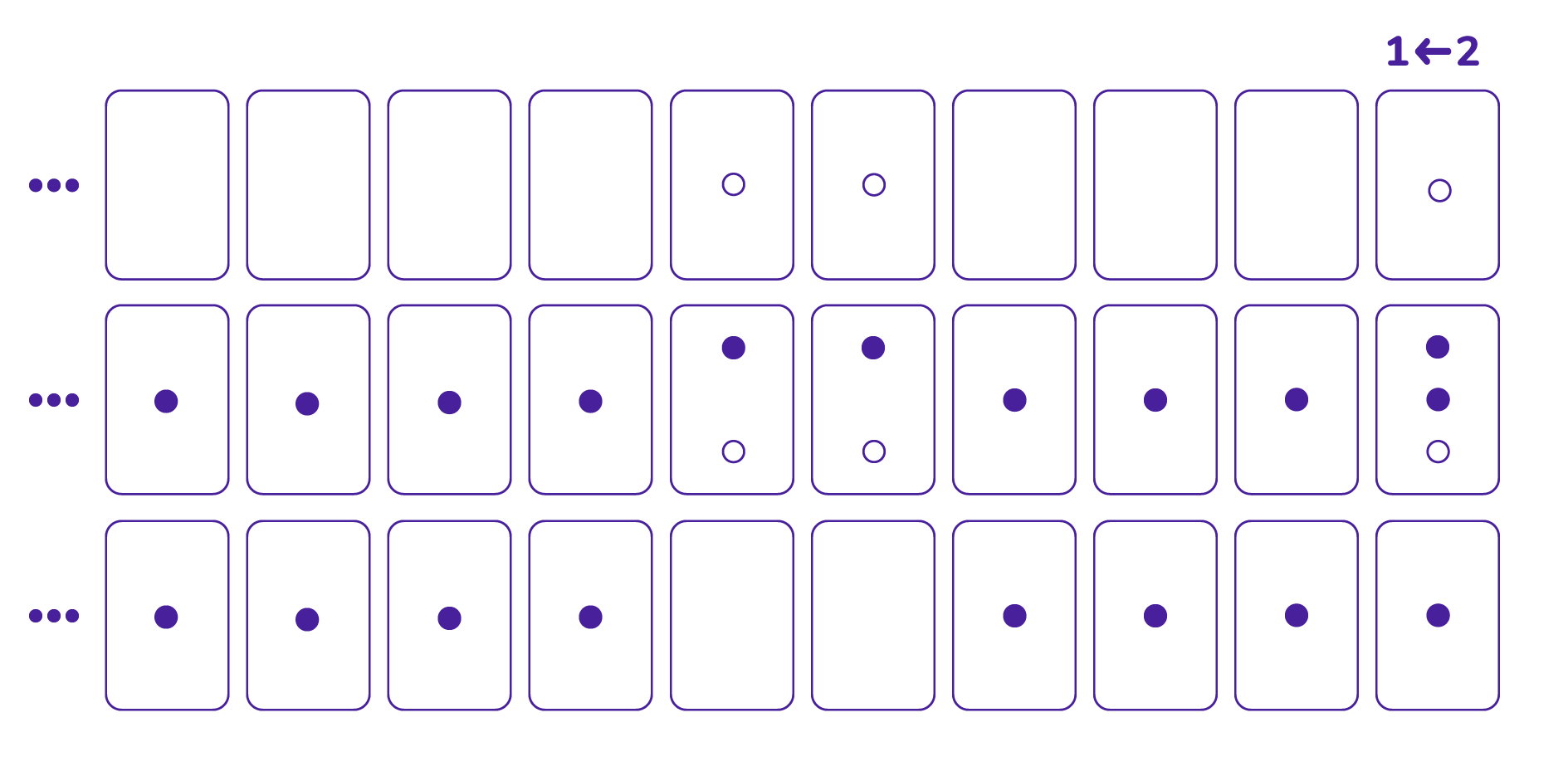Thus every negative number can be presented in a $1 \leftarrow 2$ machine without the use of any antidots. (The trade-off is that one must then use an infinite number of dots!)

Compute $106 - 49$ in Napier’s checkerboard again but this time thinking of it as an addition problem, $106 + \left(-49\right)$, that can be presented on the board using only dots.

### Let's Go Wild!